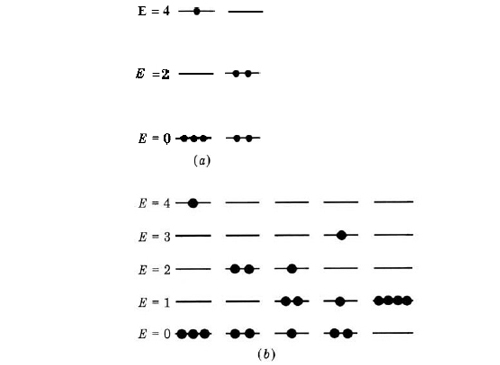# 16.7: Dependence of S on Molecular Structure

Since they all refer to the same temperature and pressure, and also to 1 mol of substance, any differences in the entropy values listed in the table of standard entropies must be due to differences in the molecular structure of the various substances listed. There are two aspects of the molecular structure of a substance which affect the value of its entropy: (1) The degree to which the movement of the atoms and molecules in the structure is restricted—the less restricted this movement, the greater the entropy. (2) The mass of the atoms and molecules which are moving—the greater the mass, the larger the entropy. We will consider each of these factors in turn.

Table $$\PageIndex{1}$$ Entropy Values

 Compound Smo /J K-1mol-1 Compound Smo /J K-1mol-1 Solids Diatomic Gases C (diamond) 2.377 C (graphite) 5.74 H2 130.7 Si 18.8 D2 145.0 Ge 31.1 HCl 186.9 Sn (gray) 44.1 HBr 198.7 Pb 64.8 HI 206.6 Li 29.1 N2 191.6 Na 51.2 O2 205.1 K 64.2 F2 202.8 Rb 69.5 Cl2 223.1 Cs 85.2 Br2 245.5 NaF 51.5 I2 260.7 MgO 26.9 CO 197.7 AlN 20.2 Triatomic Gases NaCl 72.1 H2O 188.8 KCl 82.6 NO2 240.1 Mg 32.7 H2S 205.8 Ag 42.6 CO2 213.7 I2 116.1 SO2 248.2 MgH2 31.1 N2O 219.9 AgN3 99.2 O3 238.9 Liquids Polyatomic Gases( > 3) Hg 76.0 CH4 186.3 Br2 152.2 C2H6 229.6 H2O 69.9 C3H8 269.9 H2O2 109.6 C4H10 310.2 CH3OH 126.8 C5H12 348.9 C2H5OH 160.7 C2H4 219.6 C6H6 172.8 N2O4 304.3 BCl3 206.3 B2H6 232.0 Monatomic Gases BF3 254.0 He 126.0 NH3 192.5 Ne 146.2 Ar 154.8 Kr 164.0 Xe 169.6

Restrictions on the Movement of Atoms and Molecules We have already encountered two examples of how the removal of a restriction on the motion of molecules allows an increase in the number of alternative ways in which the molecules can arrange themselves in space. The first of these is the case of the ring and chain forms of 1,4-butanediol discussed in the section on the molecular view of equilibrium, and displayed in figures 2 and 3 in that section. When the two ends of this molecule hydrogen bond to form a ring, the result is a fairly rigid structure. However, if the restricting influence of the hydrogen bond is removed, the more flexible chain form of the molecule is capable of many more alternative configurations. As a result, we find that W for a mole of molecules in the chain form is very much larger than W for a mole of molecules in the ring form. The chain form turns out to have a molar entropy which is 41 J K–1 mol–1 higher than that for the ring form.

A second example of the effect of relaxing a restriction on molecular motion is the expansion of a gas into a vacuum. When the molecules have more freedom of movement, many more alternative arrangements are possible, and hence W is larger. As we have already calculated, doubling the volume of a gas increases its molar entropy by 5.76 J K–1 mol–1.

Mass of the Atoms and Molecules We showed that when quantum mechanics is applied, the energies of a moving particle are confined to certain specific values and not those in between. The allowed energies, or energy levels, for a particle in a box were given by equation 4 in the section on wave mechanics: $E_{k}=n^{\text{2}}\frac{h^{\text{2}}}{\text{8}md^{\text{2}}}$ From this equation we can see that the larger the mass m of a particle, the smaller the value of its energy Ek for a given value of the quantum number n. Furthermore, the larger the value of m, the smaller the difference between Ek2 (for n = 2) and Ek1 (for n = 1). No matter what kind of energy is involved—rotational, vibrational, or translational—this is always found to be true. The greater the mass of a particle, the closer together its energy levels.

The effect of closeness of energy levels on the entropy is shown in Fig. $$\PageIndex{1}$$. The more closely spaced the levels, the more different ways the same quantity of energy can be distributed among them. This applies in general for any number of particles and any quantity of energy. Therefore, the heavier the molecules of a substance, the larger its molar entropy. This effect is quite obvious among the noble gases—their molar entropies increase steadily with molar (and hence molecular) mass.Figure $$\PageIndex{1}$$ Effect of molecular mass on entropy. (a) The energy levels available to light molecules are widely spaced. If the spacing is two units, and if four units of energy are supplied to four molecules, only two arrangements are possible: either one molecule has all the energy, or two molecules have two units each. (b) For heavier molecules the energy levels are closer together. If the spacing is one unit, and four units of energy are supplied, three more ways of distributing the energy are possible. Therefore the entropy is greater.